# Naming Segments, Rays, and Lines Online Quiz

#### Complete Python Prime Pack

9 Courses     2 eBooks

#### Artificial Intelligence & Machine Learning Prime Pack

6 Courses     1 eBooks

#### Java Prime Pack

9 Courses     2 eBooks

Following quiz provides Multiple Choice Questions (MCQs) related to Naming Segments, Rays, and Lines. You will have to read all the given answers and click over the correct answer. If you are not sure about the answer then you can check the answer using Show Answer button. You can use Next Quiz button to check new set of questions in the quiz.Q 1 - Identify given figure as a line segment, a ray or a line. Then name the figure using the given points.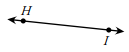### Explanation

Step 1:

The given figure is a line.

Step 2:

The line is named as HI

Q 2 - Identify given figure as a line segment, a ray or a line. Then name the figure using the given points.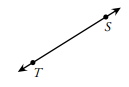### Explanation

Step 1:

The given figure is a line.

Step 2:

The line is named as TS

Q 3 - Identify given figure as a line segment, a ray or a line. Then name the figure using the given points.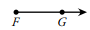### Explanation

Step 1:

The given figure is a ray.

Step 2:

The ray is named as FG.

Q 4 - Identify given figure as a line segment, a ray or a line. Then name the figure using the given points.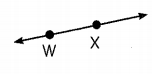### Explanation

Step 1:

The given figure is a line.

Step 2:

The line is named as WX.

Q 5 - Identify given figure as a line segment, a ray or a line. Then name the figure using the given points.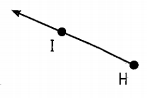### Explanation

Step 1:

The given figure is a ray.

Step 2:

The ray is named as HI.

Q 6 - Identify given figure as a line segment, a ray or a line. Then name the figure using the given points.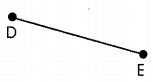### Explanation

Step 1:

The given figure is a line segment.

Step 2:

The line segment is named as DE.

Q 7 - Identify given figure as a line segment, a ray or a line. Then name the figure using the given points.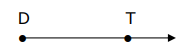### Explanation

Step 1:

The given figure is a ray.

Step 2:

The ray is named as DT.

Q 8 - Identify given figure as a line segment, a ray or a line. Then name the figure using the given points.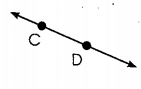### Explanation

Step 1:

The given figure is a line.

Step 2:

The line is named as CD.

Q 9 - Identify given figure as a line segment, a ray or a line. Then name the figure using the given points.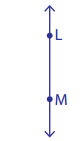### Explanation

Step 1:

The given figure is a line.

Step 2:

The line is named as LM.

Q 10 - Identify given figure as a line segment, a ray or a line. Then name the figure using the given points.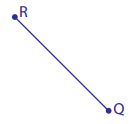### Explanation

Step 1:

The given figure is a line segment.

Step 2:

The line segment is named as RQ.

naming_segments_rays_and_lines.htm